# Diofant 2

Is equation
$70x+52y=34$
solvable on the set of integers Z?

Result

### Step-by-step explanation:

The equation is a linear Diofant equation with positive coefficients

The equation has 0 positive integer solutions

Calculated by our Diofant problems and integer equations.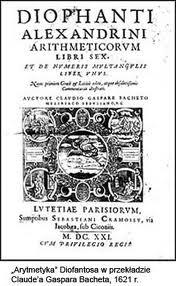Did you find an error or inaccuracy? Feel free to write us. Thank you!Tips to related online calculators
Do you want to calculate greatest common divisor two or more numbers?
Do you solve Diofant problems and looking for a calculator of Diofant integer equations?

## Related math problems and questions:

• Diofant equationIn the set of integers (Z) solve the equation: 212x +316y =0 Write result with integer parameter t in Z (parameter t = ...-2,-1,0,1,2,3... if equation has infinitely many solutions)
• Bricks pyramidHow many 50cm x 32cm x 30cm brick needed to built a 272m x 272m x 278m pyramid?
• Lcm = 22 + gcdThe least common multiple of two numbers is 22 more than their greatest common divisor. Find these numbers.
• QuotientFind quotient before the bracket - the largest divisor 51 a + 34 b + 68 121y-99z-33
• Diofant equation250x + 120y = 5640
• Four integersFnd four consecutive integers so that the product of the first two is 70 times smaller than the product of the next two.
• Seedcake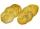Seedcake costs 44 cents. How many minimum seedcakes we must buy that we can pay in cash only whole euros?
• Gcd and lcm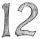Calculate the greatest common divisor and the least common multiple of numbers. a) 16 and 18 b) 24 and 22 c) 45 and 60 d) 36 and 30
• TissuesThe store got three kinds of tissues - 132 children, 156 women and 204 men. Tissues each species were packed into boxes after the number of pieces the same for all three types (and greatest). Determine the number, if you know that every box has more than
• SegmentsLine segments 62 cm and 2.2 dm long we divide into equal parts which lengths in centimeters is expressed integer. How many ways can we divide?
• MO C–I–1 2018An unknown number is divisible by just four numbers from the set {6, 15, 20, 21, 70}. Determine which ones.
• Four poplarsFour poplars are growing along the way. The distances between them are 35 m, 14 m, and 91 m. At least how many poplars need to be dropped to create the same spacing between the trees? How many meters will it be?
• Common divisorsFind all common divisors of numbers 30 and 45.
• LCD 2The least common denominator of 2/5, 1/2, and 3/4
• The classroomThe classroom is 9 meters long. The width of the classroom is smaller and can be passed in equally long steps of 55 CM or 70 CM. Determine the width of the classroom.
• Chairs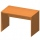In the two dining rooms in the recreational building, there are equally arranged chairs around the tables. A maximum of 78 people can dine in the first dining room and 54 people in the second. How many chairs can be around one table?
• Seamstress 2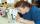Seamstress bought two kinds of textile in whole meters. One at 50 SKK and the second 70 SKK per meter. How many meter bought from this two textiles when paid totally 1540 SKK?